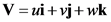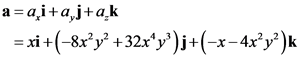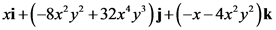# Determine the acceleration field for a three-dimensional flow with velocity components

General guidance

Concepts and Reason

Velocity of a fluid particle is a function of location and time. Velocity is a vector which has both magnitude and direction.
The acceleration of a fluid particle is the time rate of change of velocity.

Fundamentals

The velocity vector for a fluid particle,

The acceleration vector for a fluid particle in three dimensional flow,

The scalar components of acceleration vector,

Step-by-step

Step 1 of 2

The x-component of acceleration,

The y-component of acceleration,

The z-component of acceleration,

Find the components of acceleration along the three Cartesian coordinate axes using the spatial and time derivatives of velocity components.

Find the acceleration field.

Step 2 of 2

Find the acceleration field using the relation,

The acceleration field is .

Write the acceleration field vector using the components of acceleration of the fluid particle.

The acceleration field is .